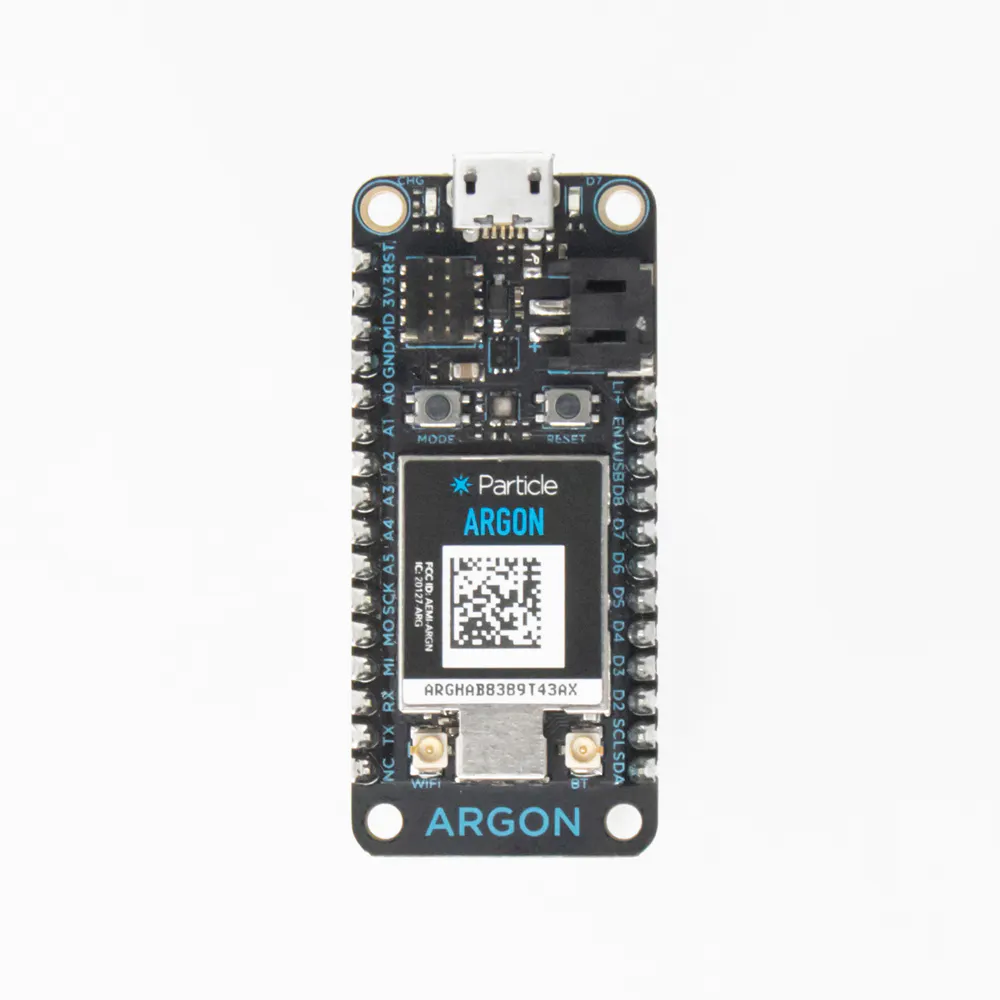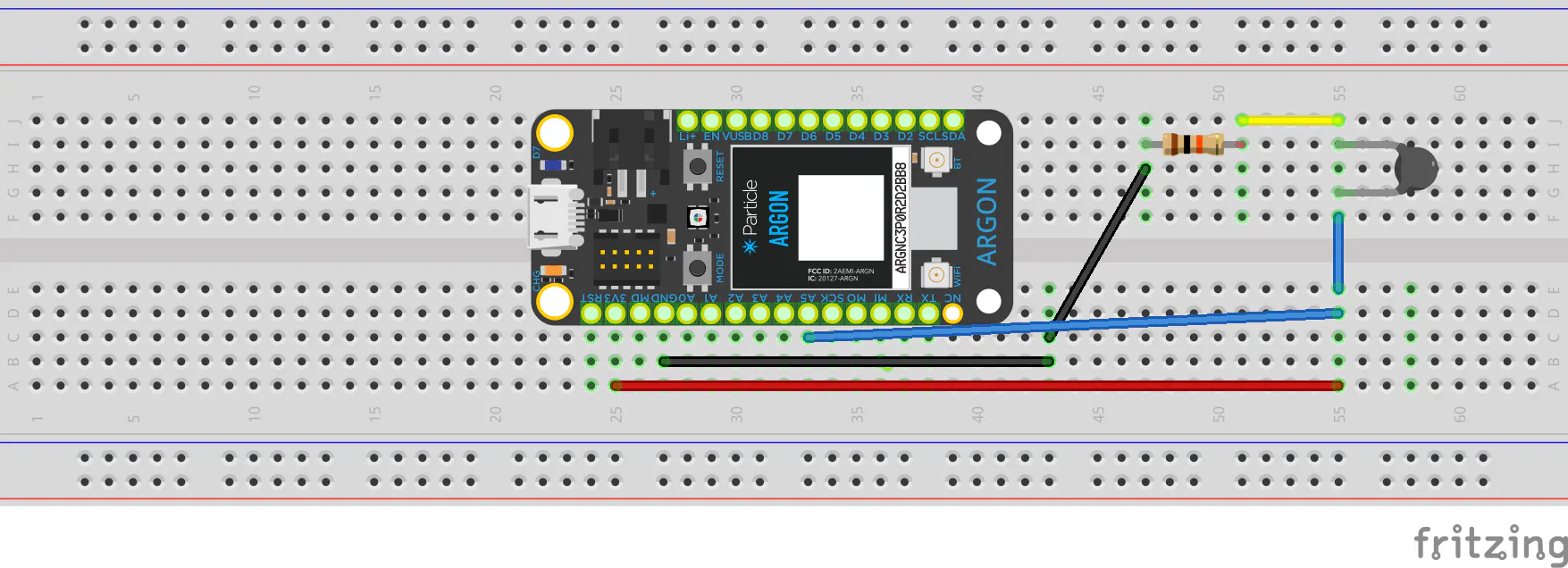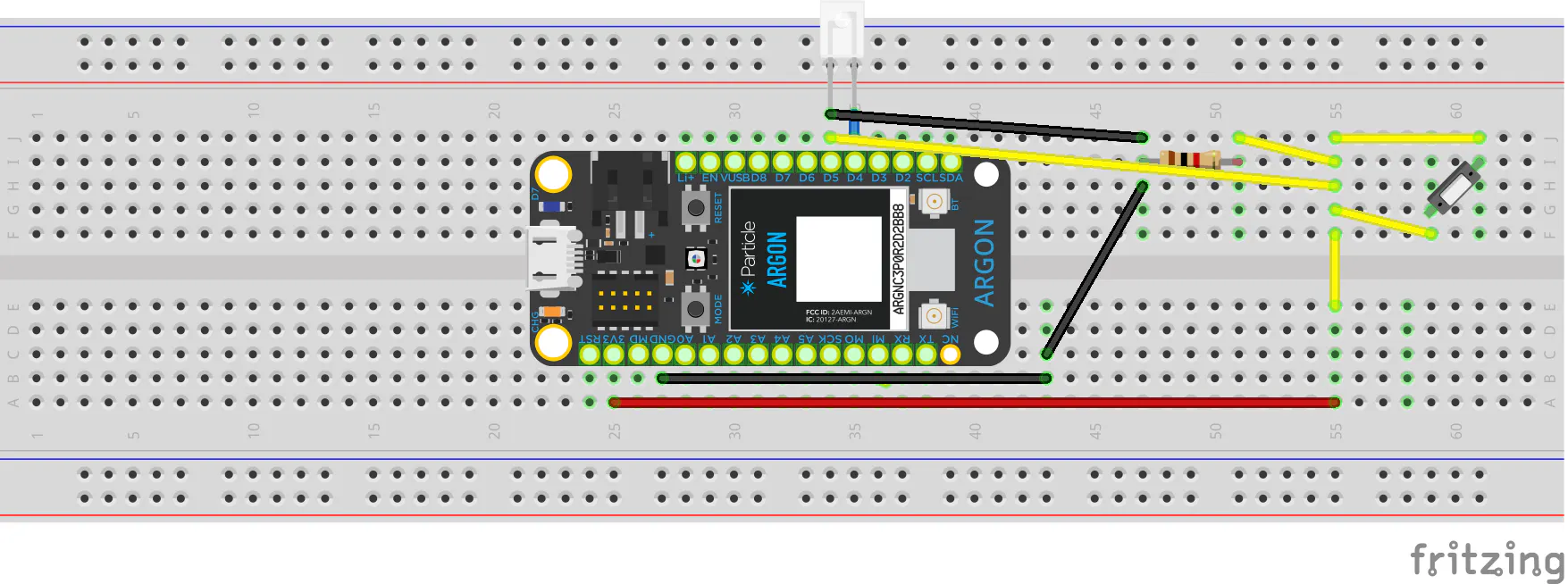Published

# Aquarium Temperature and Event Log Monitor

A Particle based system for monitoring temperature remotely with an included ant-tamper log

IntermediateShowcase (no instructions)24 hours63## Things used in this project

### Hardware componentsParticle Argon
×2Resistor 1k ohm
×1Resistor 10k ohm
×1×2
 Omron Electronic Components LLC GLS-S1 Magnetic Proximity Sensor
×1
×1

### Software apps and online servicesThingSpeak APIParticle Build Web IDE
 IFTTT

## Schematics

### Thermistor Circuit### Magnetic Sensor Circuit## Code

### Magnetic Sensor Code

C/C++
```int inputPin = D3; //defined particles inputs
int light = D4;
int val = 0;

void setup() {
pinMode(light, OUTPUT); //pin modes
pinMode(inputPin, INPUT);

}

void loop() {

if (val == HIGH){ // if loop to determine position of gate
Particle.publish("tank", "tankopen"); //publish pos if open
digitalWrite (light,HIGH);
Particle.variable("gate", 1);
delay (3000);
}
if (val == LOW){
Particle.publish("tank","tankclosed"); //publish pos if closed
digitalWrite (light,LOW);
Particle.variable("gate", 0);

delay (3000);

}

delay (1000);

}
```

### Thermistor Argon Code

C/C++
```#include <ThingSpeak.h>
#include "ThingSpeak.h"

#include <math.h> //include library for math calc

//#include <ThingSpeak_h>

TCPClient client;

int ThermPin = A5; //defining var and pin locations
int Vo;
//double RT;
//double ln;
//double VR;
double R1 = 9870; //describing var
double logR;
double R2;
double T;
double value;
double c1 = 0.001129438;// coefficents of thermistor based on known tested values and data sheet
double c2 = 0.0002333512;
double c3 = 0.0000000893741;
//const char * temp = "0";

;
void setup() {

ThingSpeak.begin(client); //int thingspeak w/ webhook
Serial.begin(9600);
pinMode(ThermPin, INPUT); //set particle inputs and output vars
Particle.variable("temp", value);

}

void loop() {

Vo = analogRead(ThermPin); //themistor equation to turn delta v into temperature in degrees C
R2 = R1*(2240/((double)Vo-1));
logR = log(R2);
T = (1.0 / (c1 + (c2 * logR) + c3 *( logR * logR * logR)));
double value = T - 273.15;

Particle.publish("value", String::format("%.4f", value));

if Particle.subscribe("gate", 1 , ALL_DEVICES){ // If gate is open particle turns indicator light on
Particle.publish( digitalWrite (light,HIGH));
}

delay(20000);
}
```

## Credits

### Thomas Nemanich

1 project • 0 followers

### Alex Mender

1 project • 0 followers